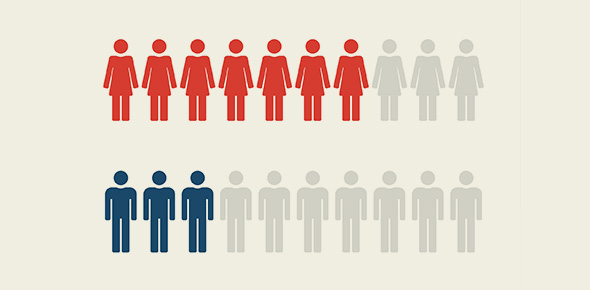# Chapter 1 : Population Quiz

5 Questions | Attempts: 86
ShareSettingsWe create this quiz is to test how well the students understand chapter 1.

• 1.
What is population ?
• A.

Population mean collection of human beings in an area.

• B.

Population mean number collection of animals.

• C.

Population mean a collection of every creatures in an area.

• D.

Population mean the amount of death per year.

• 2.
What is the formula for growth rate?
• A.

Growth Rate=crude birth rate - crude death rate.

• B.

Growth Rate=crude birth rate - net immigration rate.

• C.

Growth Rate=crude birth rate - crude death rate + net immigration rate.

• D.

Growth Rate=crude death rate + net immigration rate.

• 3.
What is population growth?
• A.

It mean number of people live in the area.

• B.

It mean number of people born.

• C.

It mean number of baby in a country.

• D.

It means a change in population over time.

• 4.
Did the population distribution evenly?
• A.

Yes.

• B.

No.

• C.

Unknown.

• 5.
What is Singapore's Population Growth Rate in 2008?
• A.

Singapore's population growth rate is 2.134%

• B.

Singapore's population growth rate is 1.135%

• C.

Singapore's population growth rate is 3.845%

• D.

Singapore's population growth rate is 0.569%

## Related TopicsBack to top
×

Wait!
Here's an interesting quiz for you.